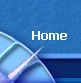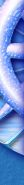SorreraSorrera | Sorrera I | Sorrera II Sorrera III | Sorrera IV Sorrera Genesis | Fisheye | Moldatu | Moldatu FullSYMMETRY OF PLATONIC SOLIDS AND THE GOLDEN MEAN Symmetry of Platonic Solids Cube & Octahedron -         They both have the same number of edges, being 12 -         The number of faces and vertices are interchanged o       Cube has 6 faces and 8 vertices o       Octahedron has 8 faces and 6 vertices Dodecahedron & Icosahedron -         They both have the same number of edges, being 30 -         The number of faces and vertices are interchanged o       Dodecahedron has 12 faces and 20 vertices o       Icosahedron has 20 faces and 12 vertices Symmetry of Mapping One Solid Into Its Reciprocal/Dual If you connect the centre of all the faces of the Cube you get the Octahedron If you connect the centre of all the faces of the Octahedron you get the Cube If you connect the centre of all the faces of the Icosahedron you get the Dodecahedron If you connect the centre of all the faces of the Dodecahedron you get the Icosahedron The Tetrahedron is self reciprocating. That is, if you join the centres of the Tetrahedrons 4 faces you get another Tetrahedron Golden Ratio The ratio of the edge lengths of 2 solids (Platonic Solids that is) embedded in one another can be expressed in terms of the Golden Ratio. That is: Phi squared over the square root of five. Icosahedron and dodecahedron are related to the Golden Ratio in more ways than one. -         12 vertices of any icosahedron can be divided into 3 groups of 4 with vertices of each group lying at corners of a Golden Rectangle (The ration of length to width of the sides of the rectangle are the Golden Ratio. -         The rectangles are perpendicular to each other and this common point if the centre of the icosahedron Also, and similarly: -         The centres of 12 pentagonal faces of the dodecahedron can be divided into 3 groups of 4. And each of these groups also forms a Golden Rectangle.

 Moldatu (2.29MB) | Moldatu full (5.77MB) Sorrera | Sorrera I | Sorrera II | Sorrera III | Sorrera IV | Sorrera-Genesis | Fisheye | Dymaxion